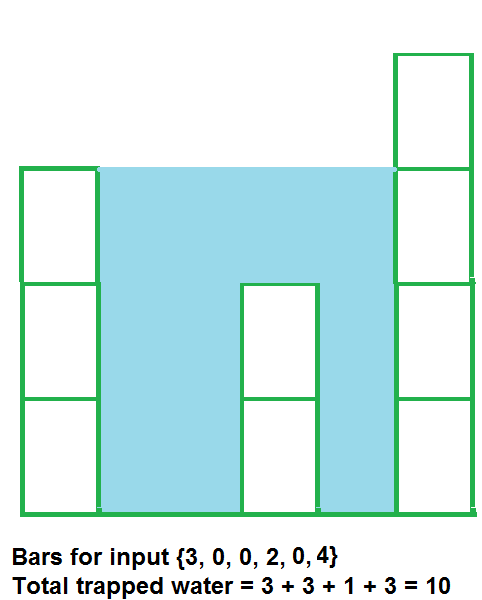XDAYS

:

HOUR

:

MINS

:

SEC

Copied to Clipboard
Trapping Rain Water
Medium Accuracy: 49.62% Submissions: 100k+ Points: 4

Given an array arr[] of N non-negative integers representing the height of blocks. If width of each block is 1, compute how much water can be trapped between the blocks during the rainy season.

Example 1:

Input:
N = 6
arr[] = {3,0,0,2,0,4}
Output:
10
Explanation:Example 2:

Input:
N = 4
arr[] = {7,4,0,9}
Output:
10
Explanation:
Water trapped by above
block of height 4 is 3 units and above
block of height 0 is 7 units. So, the
total unit of water trapped is 10 units.

Example 3:

Input:
N = 3
arr[] = {6,9,9}
Output:
0
Explanation:
No water will be trapped.

You don't need to read input or print anything. The task is to complete the function trappingWater() which takes arr[] and N as input parameters and returns the total amount of water that can be trapped.

Expected Time Complexity: O(N)
Expected Auxiliary Space: O(N)

Constraints:
3 < N < 106
0 < Ai < 108

We are replacing the old Disqus forum with the new Discussions section given below.

Editorial

We strongly recommend solving this problem on your own before viewing its editorial. Do you still want to view the editorial?

My Submissions: